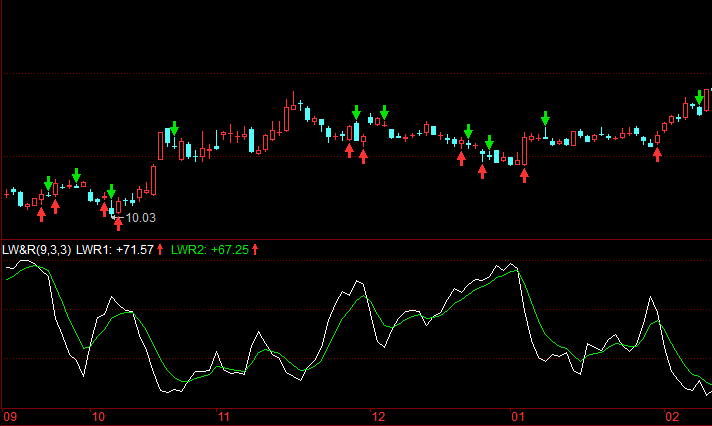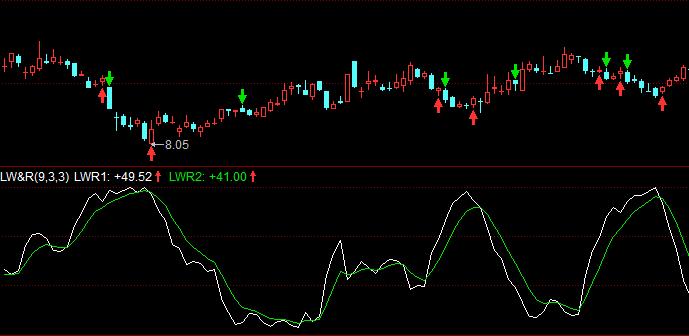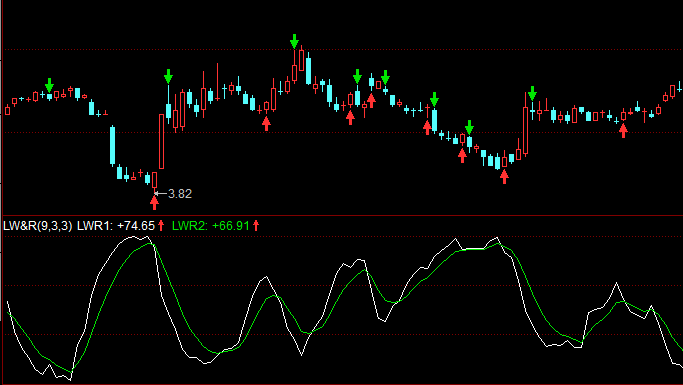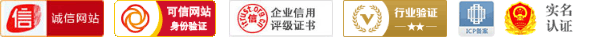## 威廉交易系统原理、用法及源代码(LW&R系统)

M1:=10;M2:=80;M3:=20;
WR:=100*(HHV(HIGH,M1)-CLOSE)/(HHV(HIGH,M1)-LLV(LOW,M1));
B:=CROSS(M2,WR);
S:=(CROSS(WR,M2)) OR (CROSS(WR,M3));
bm:=BARSLAST(b);
sm:=BARSLAST(s);
bm1:=IF(bm=-1 AND b,1,IF(bm!=-1  AND sm!=-1 AND bm>sm AND b AND b=0,1,0));
sm1:=IF(sm=-1 AND bm!=-1 AND s,1,IF( sm!=-1 AND bm!=-1 AND sm>bm AND s AND s=0,1,0));
IF(bm1>0)
IF(sm1>0)
SELL;1.高于上限，超卖，即将见底，买进信号
2.低于下限，超买，即将见顶，卖出信号

LL 下限，一般20； LH 上限，一般80

------分隔线----------------------------@2017 CFTSC 操盘手联盟工作室，欢迎您的加入，携手共创美好明天。